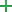# Test-Driven Development with Twisted

## Introduction

Here we'll take the tutorial "Test-Driven Development with Twisted", located at this address, and try to repeat it using PyCharm.

## Creating project, packages and Python files

On the main menu, choose File | New Project... and in the New Project dialog box, choose to create a pure Python project. Let's call it `Calculus`.

Create an empty directory called `calculus` containing an empty `__init__.py` file. To do that, choose File | New ... on the main menu, and then choose Python Package from the New pop-up menu.

Next, create a Python file. To do that, again point to File | New... on the main menu, and choose Python File in the pop-up. This file is called `base_1.py` and it opens for editing immediately. Enter the following code:

```class Calculation(object): def add(self, a, b): pass def subtract(self, a, b): pass def multiply(self, a, b): pass def divide(self, a, b): pass```

Next, right-click the package `calculus` and create a Python package again - this time it should be called `test`.

## Installing packages

You must install the Twisted package to execute tests. Besides that, if you are working on Windows, also install the package pypiwin32.

It's most easy to install the required packages in PyCharm: to do it, go to Settings/Preferences dialog and click the Project Interpreter page. On this page, in the Packages toolbar section, clickand select the required packages in the Available Packages dialog box. Read the section Installing, Uninstalling and Upgrading Packages for details.

## Selecting the required test runner

Again in the Settings/Preferences dialog, under the Tools node, click the Python Integrated Tools page.

On this page, select the test runner Twisted Trial from the list in the Default test runner field.

Apply changes and close the Settings/Preferences dialog.

## Creating a failing test

Right-click the file `base_1.py` next to the class declaration, and press Ctrl+Shift+T or choose Go To | Test on the context menu. In the Create Test Dialog you'll have to make some changes to the default settings:

First, click the browse button next to the Target directory field, and choose `test` package.

Second, in the Test file name field, enter the name `test_base_1.py`, and in the Test class name field, enter the name `TestBase_1`. Finally, select all methods - thus the tests for all the methods of the tested class will be included.

You should get the following code:

```from unittest import TestCase class TestBase_1(TestCase): def test_add(self): self.fail() def test_subtract(self): self.fail() def test_divide(self): self.fail() def test_multiply(self): self.fail()```

This is a working class, but we are writing tests for Twisted... Let's import the package. You'll end up with the following code:

```from twisted.trial import unittest class TestBase_1(unittest.TestCase): def test_add(self): self.fail() def test_subtract(self): self.fail() def test_divide(self): self.fail() def test_multiply(self): self.fail()```

As the idea of TDD (Test-Driven Development) is writing tests before the code, let's make the actual tests:

```from calculus.base_1 import Calculation from twisted.trial import unittest class CalculationTestCase(unittest.TestCase): def test_add(self): calc = Calculation() result = calc.add(3, 8) self.assertEqual(result, 11) def test_subtract(self): calc = Calculation() result = calc.subtract(7, 3) self.assertEqual(result, 4) def test_multiply(self): calc = Calculation() result = calc.multiply(12, 5) self.assertEqual(result, 60) def test_divide(self): calc = Calculation() result = calc.divide(12, 4) self.assertEqual(result, 3)```

By the way, look at the Project tool window - it shows the structure of the your project. At the moment, you have 4 files:
- `calculus/__init__.py`
- `calculus/base_1.py`
- `calculus/test/__init__.py`
- `calculus/test/test_base_1.py`

## Running the failing tests

Now let's run this test. To do that, open for editing the test file `test_base_1.py`, right-click the editor background and on the context menu choose Run 'Twisted Trial for test_base_1.CalculationTestCase'.

As expected, all tests failed:

Note that in the Project tool window appears one more directory - `_trial_temp`. It contains the log file:

## Making tests pass

Let's change the class being tested and make the tests pass. To do that, we'll change the `base_1.py` script. The code of the file will look as follows:

```class Calculation(object): def add(self, a, b): return a + b def subtract(self, a, b): return a - b def multiply(self, a, b): return a * b def divide(self, a, b): return a // b```

Let's run `test_base_1.py` again. To do it, right-click the editor background and choose Run 'Twisted Trial for test_base_1.CalculationTestCase'. This time the tests pass:

## Summary

Let us recall what we have done with the help of PyCharm:

• Installed required packages.
• Created a project, Python packages and files.
• Created tests.
• Ran the tests in the Test Runner.